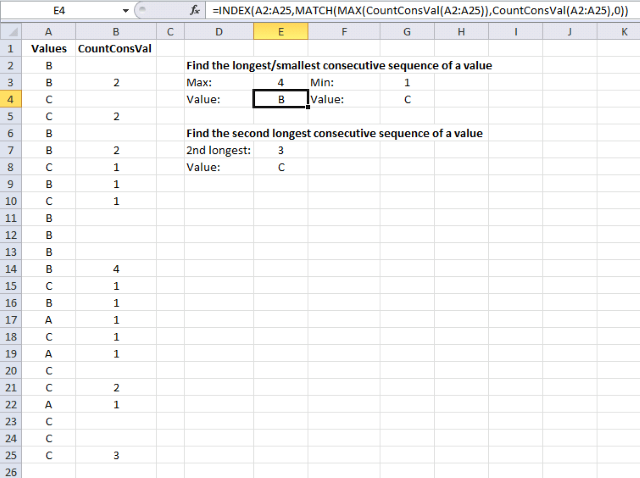Author: Oscar Cronquist Article last updated on January 14, 2018

This post Find the longest/smallest consecutive sequence of a value has a few really big array formulas. Today I would like to show you how to build a simple udf that will simplify these formulas significantly.

The following user defined function returns the size of each consecutive sequence in a range. The udf is entered in cell range B2:B25, see this picture.

The udf returns an array with the same size as the argument range. The cell range has 24 cells and the array has 24 values.

### VBA code

```
Function CountConsVal(r As Range)
Dim i As Long, s As Long
Rng = r.Value
For i = LBound(Rng, 1) To UBound(Rng, 1) - 1
If Rng(i, 1) = Rng(i + 1, 1) Then
s = s + 1
Rng(i, 1) = ""
Else
Rng(i, 1) = s + 1
s = 0
End If
Next i
Rng(UBound(Rng), 1) = s + 1
CountConsVal = Rng
End Function

```

###Array formula in cell E3:

=MAX(CountConsVal(A2:A25))

Array formula in cell E4:

=INDEX(A2:A25,MATCH(MAX(CountConsVal(A2:A25)),CountConsVal(A2:A25),0))

Array formula in cell G3:

=MIN(CountConsVal(A2:A25))

Array formula in cell G4:

=INDEX(A2:A25,MATCH(MIN(CountConsVal(A2:A25)),CountConsVal(A2:A25),0))

Array formula in cell E7:

=LARGE(CountConsVal(A2:A25),2)

Array formula in cell E8:

=INDEX(A2:A25,MATCH(LARGE(CountConsVal(A2:A25),2),CountConsVal(A2:A25),0))

### Build a user defined function

To build a user defined function, follow these steps:

1. Press Alt + F11 to open the visual basic editor
2. Click "Insert" on the menu
3. Click "Module"4. Copy the code above and paste it to the code module.

### Explaining the user defined function

Function name and arguments

A user defined function procedure always start with "Function" and then a name. This udf has a single argument r. Variable r is a range.

```Function CountConsVal(r As Range)
```

Declaring variables

Variable i and s are declared data type Long. Read more about Defining data types.

```Dim i As Long, s As Long
```

Transfer values from r (range) to a Rng (variant) array

```Rng = r.Value
```

For ... Next statement

Repeats a group of statements a specified number of times. LBound returns the lower bound of an array and UBound the upper bound.

LBound(array, dimension) UBound(array, dimension), the dimension arguemnt can be omitted if the array is a one dimensional array. In this case the Variant Rng is a two dimensional array despite the fact that the range r is a one dimensional array. UBound(Rng, 1) returns the number of rows in Rng array.

```For i = LBound(Rng, 1) To UBound(Rng, 1) - 1

...

Next i
```

If ... then... Else ... End If

Check if the current value (i) is equal to the next value (i+1)

```If Rng(i, 1) = Rng(i + 1, 1) Then
```

Add number 1 to variable s

```s = s + 1
```

Delete value in array

```Rng(i, 1) = ""
```

Assign a value (s + 1) to array variable Rng(i, 1)

The current value in the array is equal to s + 1

```Rng(i, 1) = s + 1
```

Assign 0 (zero) to variable s

```s = 0
```

Assign a value (s + 1) to the last value in the array Rng(UBound(Rng), 1)

```Rng(UBound(Rng), 1) = s + 1
```

The udf returns an array of values

```CountConsVal = Rng
```

End a udf

A function procedure ends with this statement.

```End Function
```

### Download excel * .xlsm file

Longest-consecutive-sequence.xlsm